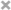跟我一起写Makefile
+ -

# makefile 函数

2023-03-09 3 0

## 函数的调用语法

``````\$(<function> <arguments>)
``````

``````\${<function> <arguments>}
``````

``````comma:= ,
empty:=
space:= \$(empty) \$(empty)
foo:= a b c
bar:= \$(subst \$(space),\$(comma),\$(foo))
``````

## 字符串处理函数

#### ### subst

``````\$(subst <from>,<to>,<text>)
``````

``````\$(subst ee,EE,feet on the street)
``````

#### patsubst

``````\$(patsubst <pattern>,<replacement>,<text>)
``````

``````\$(patsubst %.c,%.o,x.c.c bar.c)
``````

``````objects = foo.o bar.o baz.o，
``````

#### strip

``````\$(strip <string>)
``````

``````\$(strip a b c )
``````

#### findstring

``````\$(findstring <find>,<in>)
``````

``````\$(findstring a,a b c)
\$(findstring a,b c)
``````

#### filter

``````\$(filter <pattern...>,<text>)
``````

``````sources := foo.c bar.c baz.s ugh.h
foo: \$(sources)
cc \$(filter %.c %.s,\$(sources)) -o foo
\$(filter %.c %.s,\$(sources)) 返回的值是 foo.c bar.c baz.s 。
``````

#### filter-out

``````\$(filter-out <pattern...>,<text>)
``````

``````objects=main1.o foo.o main2.o bar.o
mains=main1.o main2.o
\$(filter-out \$(mains),\$(objects)) 返回值是 foo.o bar.o 。
``````

#### sort

``````\$(sort <list>)
``````

#### word

``````\$(word <n>,<text>)
``````

#### wordlist

``````\$(wordlist <ss>,<e>,<text>)
``````

#### words

``````\$(words <text>)
``````

#### firstword

``````\$(firstword <text>)
``````

``````override CFLAGS += \$(patsubst %,-I%,\$(subst :, ,\$(VPATH)))
``````

## 文件名操作函数

#### dir

``````\$(dir <names...>)
``````

#### notdir

``````\$(notdir <names...>)
``````

#### suffix

``````\$(suffix <names...>)
``````

#### basename

``````\$(basename <names...>)
``````

``````\$(addsuffix <suffix>,<names...>)
``````

``````\$(addprefix <prefix>,<names...>)
``````

#### join

``````\$(join <list1>,<list2>)
``````

#### foreach 函数

foreach函数和别的函数非常的不一样。因为这个函数是用来做循环用的，Makefile中的foreach函数几乎是仿照于Unix标准`Shell（/bin/sh）`中的for语句，或是C-Shell（/bin/csh）中的foreach语句而构建的。它的语法是：

``````\$(foreach <var>,<list>,<text>)
``````

``````names := a b c d

files := \$(foreach n,\$(names),\$(n).o)
``````

#### if 函数

if函数很像GNU的make所支持的条件语句——ifeq（参见前面所述的章节），if函数的语法是：

``````\$(if <condition>,<then-part>)
``````

``````\$(if <condition>,<then-part>,<else-part>)
``````

#### call函数

call函数是唯一一个可以用来创建新的参数化的函数。你可以写一个非常复杂的表达式，这个表达式中，你可以定义许多参数，然后你可以call函数来向这个表达式传递参数。其语法是：

``````\$(call <expression>,<parm1>,<parm2>,...,<parmn>)
``````

``````reverse =  \$(1) \$(2)

foo = \$(call reverse,a,b)
``````

``````reverse =  \$(2) \$(1)

foo = \$(call reverse,a,b)
``````

#### origin函数

origin函数不像其它的函数，他并不操作变量的值，他只是告诉你你的这个变量是哪里来的？其语法是：

``````\$(origin <variable>)
``````

\$ 字符。Origin函数会以其返回值来告诉你这个变量的“出生情况”，下面，是origin函数的返回值:

undefined

default

environment

file

command line

override

automatic

``````ifdef bletch
ifeq "\$(origin bletch)" "environment"
bletch = barf, gag, etc.
endif
endif
``````

#### shell函数

shell函数也不像其它的函数。顾名思义，它的参数应该就是操作系统Shell的命令。它和反引号“`”是相同的功能。这就是说，shell函数把执行操作系统命令后的输出作为函数返回。于是，我们可以用操作系统命令以及字符串处理命令awk，sed等等命令来生成一个变量，如：

``````contents := \$(shell cat foo)
files := \$(shell echo *.c)
``````

## ## 控制make的函数

make提供了一些函数来控制make的运行。通常，你需要检测一些运行Makefile时的运行时信息，并且根据这些信息来决定，你是让make继续执行，还是停止。

``````\$(error <text ...>)
``````

``````ifdef ERROR_001
\$(error error is \$(ERROR_001))
endif
``````

``````ERR = \$(error found an error!)

.PHONY: err

err: \$(ERR)
``````

``````\$(warning <text ...>)
``````

#### 0 篇笔记 写笔记

makefile概述什么是makefile？或许很多Windows的程序员都不知道这个东西，因为那些Windows的集成开发环境（integrated development environment，IDE）都为你做了这个工作，但我觉得要作一个好的和专业的程序员，makefile还是要懂。这就好像现......
makefile介绍make命令执行时，需要一个makefile文件，以告诉make命令需要怎么样的去编译和链接程序。首先，我们用一个示例来说明makefile的书写规则，以便给大家一个感性认识。这个示例来源于gnu 的make使用手册，在这个示例中，我们的工程有8个c文件，和3个头文件，我们要写......Windows驱动开发，网站开发